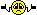<![CDATA[Math Is Fun Forum / radius & coordinates]]> 2016-12-18T00:53:38Z FluxBB https://www.mathisfunforum.com/viewtopic.php?id=5039 <![CDATA[Re: radius & coordinates]]> bob bundy wrote:

hi Monox D. I-Fly

Looks to me like that poster just made a mistake clearing the brackets.  Should be:

There's a great page on this here:

http://www.mathsisfun.com/algebra/circle-equations.html

Bob

Well, since no one has responded in 10 years...

bob bundy wrote:

ps.  unique hasn't posted since 27th Feb 2007 so it's unlikely you'll get a response from the poster.  People join, post for a while, and then stop.  If you find an old post like this one, it's worth clicking the poster's name and then their posts to see when the last one was.  That way you'll know if they are continuing to be an active member.

Even though he isn't here anymore, we can still help others who read this post and want answers, no?

]]>
https://www.mathisfunforum.com/profile.php?id=212031 2016-12-18T00:53:38Z https://www.mathisfunforum.com/viewtopic.php?pid=391673#p391673
<![CDATA[Re: radius & coordinates]]> hi Monox D. I-Fly

Looks to me like that poster just made a mistake clearing the brackets.  Should be:

There's a great page on this here:

http://www.mathsisfun.com/algebra/circle-equations.html

Bob

ps.  unique hasn't posted since 27th Feb 2007 so it's unlikely you'll get a response from the poster.  People join, post for a while, and then stop.  If you find an old post like this one, it's worth clicking the poster's name and then their posts to see when the last one was.  That way you'll know if they are continuing to be an active member.

]]>
https://www.mathisfunforum.com/profile.php?id=67694 2016-12-17T08:55:01Z https://www.mathisfunforum.com/viewtopic.php?pid=391571#p391571
<![CDATA[Re: radius & coordinates]]> unique wrote:

so in our problem:
(x - a)^2 + (y - b)^2 = r^2 right
so we can do teh same way like the way they did in the book?
like
x^2 - a^2 + y^2 - b^2 + ay - ab = r^2 then what i think i have the wrong way in doing this...help

Where did you get that ay and -ab from? (x - a)^2 is x^2 - 2ab + a^2.

]]>
https://www.mathisfunforum.com/profile.php?id=212031 2016-12-16T08:41:37Z https://www.mathisfunforum.com/viewtopic.php?pid=391529#p391529
<![CDATA[Re: radius & coordinates]]> hello help anyone?

]]>
https://www.mathisfunforum.com/profile.php?id=5209 2006-11-15T16:39:37Z https://www.mathisfunforum.com/viewtopic.php?pid=49145#p49145
<![CDATA[Re: radius & coordinates]]> ok i know what you are saying...
you have given me the standard circle form which i can do
which i see in the book.
but in teh book we have numbers instead of letters..can i change teh letter into number and letters back again for me to do this?

liek in the book
the standard form is > (x + 3)^2 + (y - 3)^2 = 16
then they did
x^2 + 6x + 9 + y^2 - 6y + 9 = 16
and finished by collecting like terms
x^2 + y^2 + 6x - 6y + 2 = 0

so in our problem:
(x - a)^2 + (y - b)^2 = r^2 right
so we can do teh same way like the way they did in the book?
like
x^2 - a^2 + y^2 - b^2 + ay - ab = r^2 then what i think i have the wrong way in doing this...help

]]>
https://www.mathisfunforum.com/profile.php?id=5209 2006-11-14T21:35:26Z https://www.mathisfunforum.com/viewtopic.php?pid=49107#p49107
<![CDATA[Re: radius & coordinates]]> Divide everything by 9 and group the x and y terms: x² - 2x + y² + 4y - 4 = 0

Complete the square for x and y: [(x-1)² - 1] + [(y+2)² - 4] - 4 = 0

Combine the constants: (x-1)² + (y+2)² = 3²

And there you have your standard circle form: (x-a)² + (y-b)² = r².

Can you work out the radius and centre coordinates now?

]]>
https://www.mathisfunforum.com/profile.php?id=641 2006-11-14T16:35:04Z https://www.mathisfunforum.com/viewtopic.php?pid=49083#p49083
<![CDATA[radius & coordinates]]> find the radius and coordinates of the center of the circle whose equation is

9x^2 + 9y^2 - 18x + 36y - 36 = 0

ok how do i do this...]]>
https://www.mathisfunforum.com/profile.php?id=5209 2006-11-14T14:55:15Z https://www.mathisfunforum.com/viewtopic.php?pid=49080#p49080